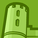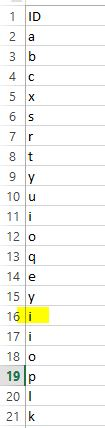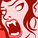QlikView App Dev

Discussion Board for collaboration related to QlikView App Development.

Announcements
QlikWorld 2022, LIVE in Denver CO., May 16-19, 2022. REGISTER NOW TO RECEIVE EARLY BIRD PRICING
cancel
Showing results for
Did you mean:Creator

Set variable from the load table

Hello Users,

I am trying to set the variable from the excel table which is loaded.

The value of the variable is set based on the condition that it should pick the value of 0.75 of the total rows.

For example

Based on the attached excel sheet, value of the variable should be 'i' as it is the 0.75 of total rows from the excel (total rows=20, 0.75 of total row is 15(=20*.75=15),so 15th row of the excel sheet is 'i' from column ID.

Need to the set the variable value in load script not in chart.1 Solution

Accepted SolutionsMVP

Try like:

Tab:

ID,

RowNo() as RowNum

FROM

[Variable.xlsx]

(ooxml, embedded labels, table is Sheet1);

Let vNoOfRows=NoOfRows('Tab');

Let v75RowNum=FieldValue('RowNum', vNoOfRows*.75);

Let v75Value=Lookup('ID','RowNum', v75RowNum);

3 RepliesMVP

Try like:

Tab:

ID,

RowNo() as RowNum

FROM

[Variable.xlsx]

(ooxml, embedded labels, table is Sheet1);

Let vNoOfRows=NoOfRows('Tab');

Let v75RowNum=FieldValue('RowNum', vNoOfRows*.75);

Let v75Value=Lookup('ID','RowNum', v75RowNum);Creator III

Hi,

Temp:

RowNo() as RowNumber

FROM

[Variable.xlsx]

(ooxml, embedded labels, table is Sheet1)

;

let vNofrows=NoOfRows('Temp')*0.75;

LET Row= peek('ID', \$(vNofrows)-1,'Temp');

Thanks,

PoojaContributor

The "peek" function solved my issue. I've been trying to figure out how to dynamically write qvds using a value in a table and add a date to the end.Community Browser